#### Welcome to Synthiam!

The easiest way to program the most powerful robots. Use technologies by leading industry experts. ARC is a free-to-use robot programming software that makes servo automation, computer vision, autonomous navigation, and artificial intelligence easy.Resolved by Nink!

## Which Way Should I Go For Spot Micro?

I have completed the mechanical assembly of Spot Micro.    There are several versions for the controlling mechanisms used by various people.  Here is a very cute one that is done with an Arduino Mega as the controller with embedded code in the Arduino.   However, I have other options too such as either using a EZB V4 or a RPI with a servo Module and some reverse kinematics software.

If I decide to use the EZB V4, this is not that different than the "Six" robot in complexity (maybe).   I have the parts and I am trying to determine which way to go.   The kicker will be designing each step of movement with frames using ARC.  I have not tried anything this complex control-wise.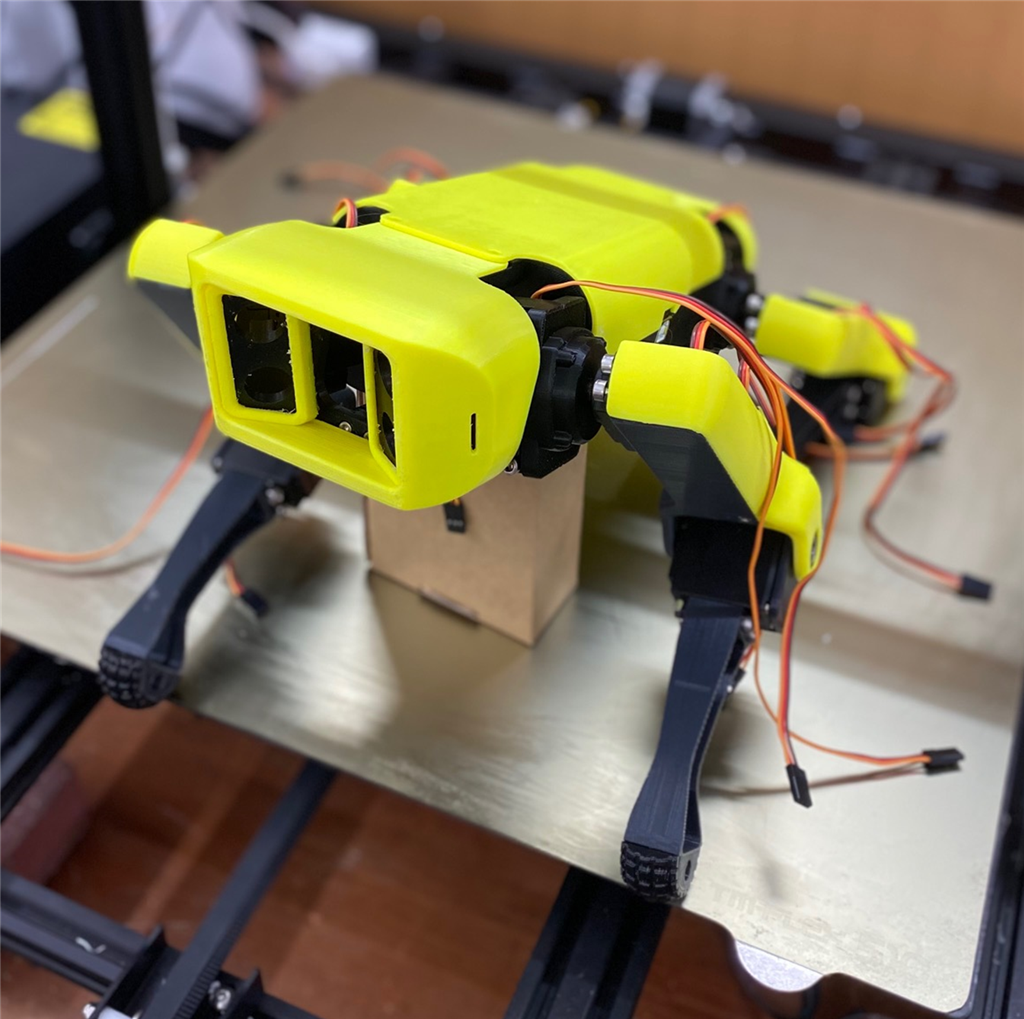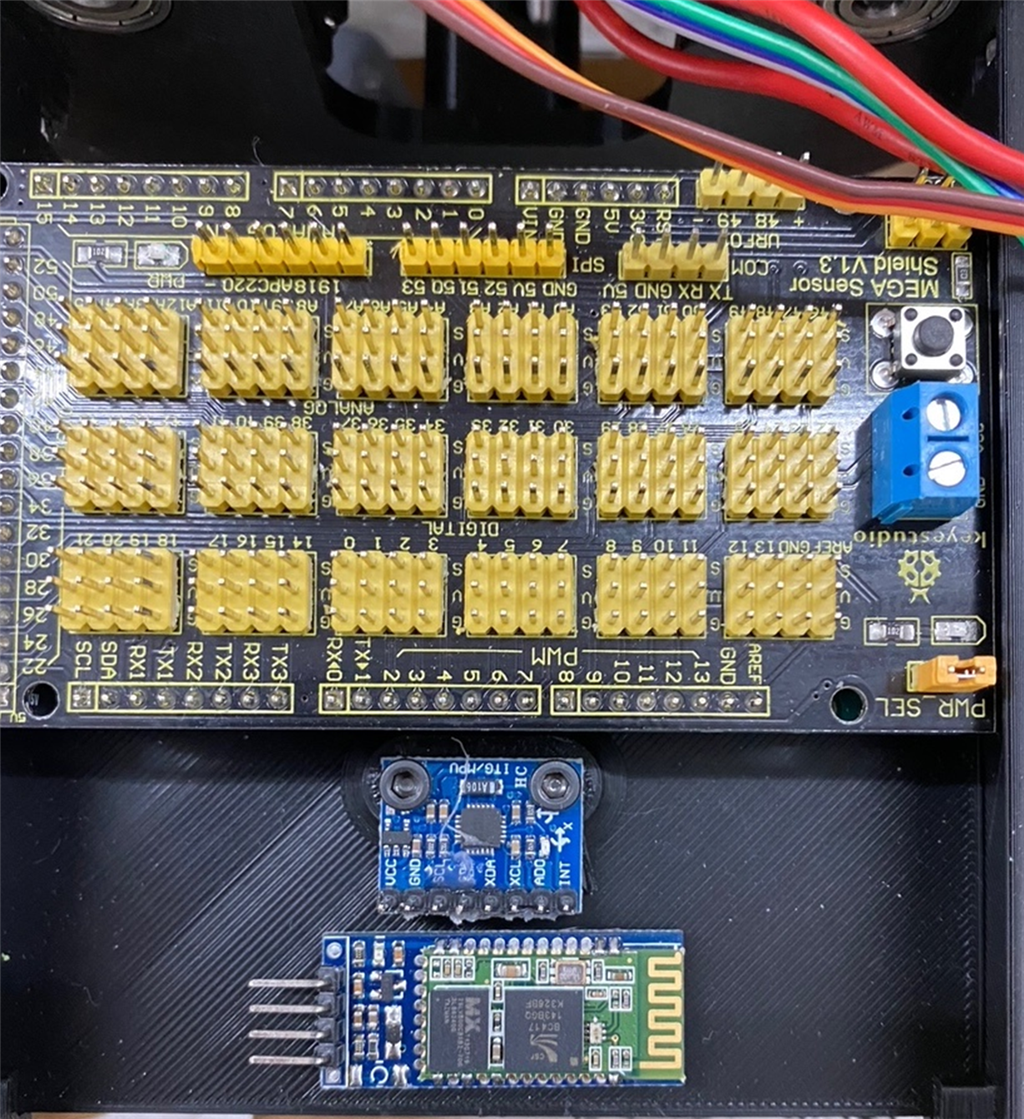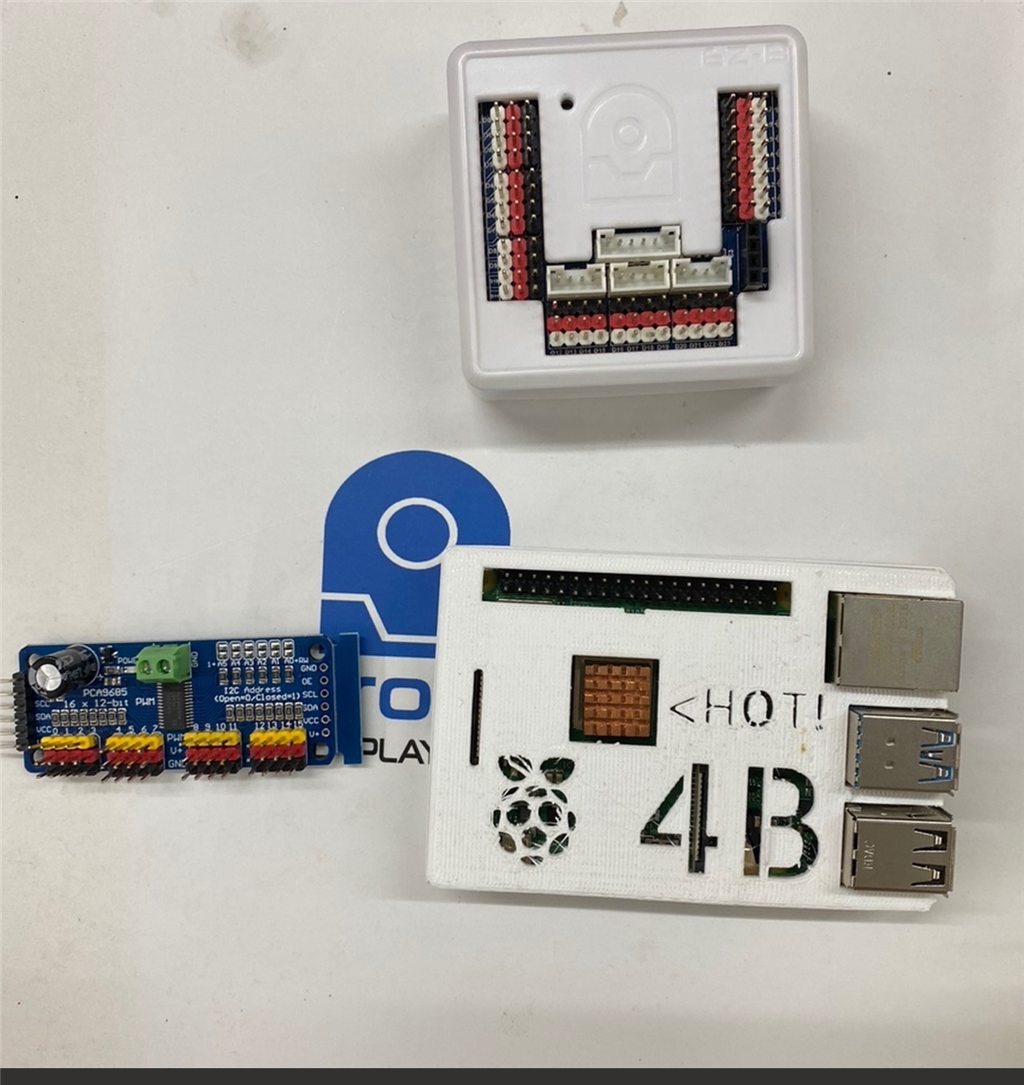Related Hardware Arduino Due/Mega

Harnessing the power of ARC Pro, your robot can be more than just a simple automated machine.Related Content
Synthiam
Based on your post activity, we found some content that may be interesting to you. Explore these other tutorials and community conversations.
#5
Cool.  I think I have another io tiny as well I could use instead of the EZB V4. Thanks for you help DJ!
#6
I’ll take a better look at the code and see what the commands are
#7   — Edited
hi all

this guy is making one .maybe is something for you?

#8
DJ,
Did you have a chance to look at the Code to see how I/we can implement the hooks?  Once I get this one done, I would like to offer it up as a spotlight for you.   Any guidance or examples that you can provide would be great.  It seems to me that what I want to do is have the ability to mimic the bluetooth connections and controls via the PS2 joystick via the IOtiny.

Also, I want to be able to read ultra sonic sensor data either from the Arduino/IO tiny.   I would like to eventually integrate a camera too in the nose- that would be direct to the IO tiny.   How do you connect the IO Tiny to the Arduino?

I will build a mobile screen app on top of it all using ARC.
#9   — Edited
DJ,
Here is INO file for Max (Spot Micro) that I translated some comments from Dutch to English where needed.   It appears this approach uses 4 analog inputs on the Arduino from a RC Controller receiver Output.  I am wondering if one setup the IO Tiny to output  4 PWM signal outputs if it could simulate the RC receiver outputs and use the same code base in the Arduino Mega.`Code:Spot Robot controllerJan De Coster07/01/2021More info on www.jandecoster.comThis code is part of an online course. Using this code on your robot requires proper knowledge of the platform.Do not forget to calibrate your robot to avoid catastrophic failureRC read based on Kelvin Nelson's example 24/07/2019https://create.arduino.cc/projecthub/kelvineyeone/read-pwm-decode-rc-receiver-input-and-apply-fail-safe-6b90eb*/#include //RC stuff//RC controller pinsconst int CH_0_PIN = A0;const int CH_1_PIN = A1;const int CH_2_PIN = A2;const int CH_3_PIN = A3;const int deadzone = 20; //center stick when close to centerint ch_0;int ch_1;int ch_2;int ch_3;//End RC stuff//Gyro stuff#include const int MPU = 0x68; // MPU6050 I2C addressfloat AccX, AccY, AccZ;float GyroX, GyroY, GyroZ;float accAngleX, accAngleY, gyroAngleX, gyroAngleY, gyroAngleZ;float roll, pitch, yaw, oldroll, oldpitch, oldyaw;float AccErrorX, AccErrorY, GyroErrorX, GyroErrorY, GyroErrorZ;float elapsedTime, currentTime, previousTime;int c = 0;bool safetymode = false;//End Gyro stuffServo servo; //array of servo'sconst int servo_pin = {2, 3, 4, 5, 6, 7, 8, 9, 10, 11, 12, 13}; //arrays of servo pinsconst int directions = {1, -1,-1, 1 ,1,1, -1 ,1,1, -1,-1,-1}; //reflection correction for opposite sides of the robotconst int base = {90 ,100 ,102 , 90 ,88 ,97 , 90.00 ,78 ,95 , 95 ,108 ,100 }; //callibration these are the base values for each servo, that put Spot in a comfortable starting position/* Servo's can not keep their current position, so we store these in an array angleCurrent * The target of each servo is placed in angleTarget, and angleSpeed holds each change in angle for each servo, per function call * arcPos holds different poses , with values relative to the base values in array base */float angleCurrent = {base, base, base, base, base, base, base, base, base, base, base, base}; //real-time arcsfloat angleTarget = {base, base, base, base, base, base, base, base, base, base, base, base}; //expected coordinates of the end of the legfloat angleSpeed = {0.00, 0.00, 0.00, 0.00, 0.00, 0.00, 0.00, 0.00, 0.00, 0.00, 0.00, 0.00}; //each axis' speed, needs to be recalculated before each movement//step sequence forwardint sb1 = -10; int so1 = 22; //sb upperarm so lowerarmint sb2 = 2; int so2 = 1;int sb3 = -9; int so3 = 1; //only for 4 step movesint sb4 = -21; int so4 = 19;//intertia compensation after runningint brb = 1; int bro = -12;//brace animation when stopping, to prevent tipping overint brcb = -14; int brco = 24;int pootb = 53; int pooto = -16;float arcPos = { //2D array with positions for each servo, adjust initialization as you add steps! //Leg Left Front------Leg right Front-----Leg Left rear----Leg Left rear // 0 //base stand {0, 0, 0, 0, 0, 0, 0, 0, 0, 0, 0, 0}, //shldr up down shldr up down shldr up down shldr up down // 1 //lay down, safety position when RC is lost {0, 0, 45, 0, 0, 45, 0, 0, 45, 0, 0, 45}, //shldr up down shldr up down shldr up down shldr up down // 2 //stand back , rease back {0, 0, 45, 0, 0, 45, 0, 0, 0, 0, 0, 0}, //shldr up down shldr up down shldr up down shldr up down // 3 //sit up {0, 0, -15, 0, 0, -15, 0, 0, 25, 0, 0, 25}, //shldr up down shldr up down shldr up down shldr up down // 4 //step 1 {0, sb1, so1, 0, sb2, so2, 0, sb1, so1, 0, sb2, so2}, //shldr up down shldr up down shldr up down shldr up down // 5 //step 2 {0, sb2, so2, 0, sb1, so1, 0, sb2, so2, 0, sb1, so1}, //shldr up down shldr up down shldr up down shldr up down // 6 //step 3 {0, sb3, so3, 0, sb1, so1, 0, sb3, so3, 0, sb1 , so1 }, //shldr up down shldr up down shldr up down shldr up down // 7 //step 4 {0, sb4, so4, 0, sb2, so2, 0, sb3, so3, 0, sb2, so2}, //shldr up down shldr up down shldr up down shldr up down // 8 //stand turn {0, 0, 0, 0, 0, 0, 0, 0, 0, 0, 0, 0}, //shldr up down shldr up down shldr up down shldr up down // 9 //break forward {0, 0, 0, 0, 0, 0, 0, sb1/3, so1/3, 0, sb1/3, so1/3}, //shldr up down shldr up down shldr up down shldr up down // 10 //break backward {0, 0, 0, 0, 0, 0, 0, brb, bro, 0, brb, bro }, //shldr up down shldr up down shldr up down shldr up down // 11 //brace {0, brcb, brco, 0, brcb, brco, 0, brcb, brco, 0, brcb, brco}, //shldr up down shldr up down shldr up down shldr up down // 12 //sniff {0, brcb, brco, 0, brcb, brco, 0, 0, 0, 0, 0, 0}, //shldr up down shldr up down shldr up down shldr up down // 13 //raise {0, 0, 0, 0, 0, 0, 0, brcb, brco, 0, brcb, brco} //shldr up down shldr up down shldr up down shldr up down }; bool to_sit = false; //is Spot sitting down?bool moving = false; //is Spot moving? then we should waitbool to_stand = false; //is Spot standing?bool movingforward = false; //is Spot moving forward?bool movingbackward = false; //is Spot moving backward?bool jumped = false; //did Spot jump?bool sniffing = false; //is Spot sniffing?bool raised = false; //is Spot looking up?int angle_of_rotation = 0; //left rightint tilt_angle = 0; // up downint fspeed = 0; //speedint currentstep = 1;void setup() { Serial.begin(9600); Wire.begin(); // Initialize comunication Wire.beginTransmission(MPU); // Start communication with MPU6050 // MPU=0x68 Wire.write(0x6B); // Talk to the register 6B Wire.write(0x00); // Make reset - place a 0 into the 6B register Wire.endTransmission(true); //end the transmission Serial.println("Gyro"); calculate_IMU_error(); delay(20); Serial.println("relay"); //pinMode(1, OUTPUT); //1 when pin available connect this to a relay for extra safety //digitalWrite(1, LOW); delay(500); Serial.println("attach"); servo_attach(); setTargets(1,10); //go to lay down, without filling target arrays //digitalWrite(1, HIGH); //start motors with relay delay(1000); Serial.println("set"); setTargets(1,10); //go to lay down to_sit = true; delay(1000); }void loop() {checkRC(); //read RCreadGyro();if(!safetymode){angle_of_rotation = ch_0/40;//angle of rotationfspeed = -ch_1/25;tilt_angle = ch_3/20; //tilt angleforwardarray();turnarray(); //walking? switch step 1 and 2 if(abs(ch_1)>40 && !to_sit && currentstep==1){ if(ch_1>0){ movingforward = true; }else{ movingbackward = true; } currentstep = 2; setTargets(4,10); } else if(abs(ch_1)>40 && !to_sit && currentstep==2){ if(ch_1>0){ movingforward = true; }else{ movingbackward = true; } currentstep = 1; setTargets(5,10); } //not walking ? else{ if(ch_2>0){ //goes channel 2 above 0? if(to_sit){ //Spot sits? standm to_sit is to sit mind_lay_down(); mind_standup(); to_sit = false; } else{ //Spot not sitting? go to stand to_sit = false; if(abs(angle_of_rotation)>2 && !to_sit){ setTargets(8,10); }else{ if(movingforward){ setTargets(9,5); movingforward = false; } else if(movingbackward){ setTargets(10,5); movingbackward = false; } mind_stand(); } } } if(ch_2<-100 && !to_sit){ //goes channel 2 below -100? to_sit = true; mind_sit(); // Spot sit } else if(ch_2<-200 && to_sit){ //channel 2 goes below -200? mind_lay_down(); //Spot lay down } else if(ch_2>220 && !jumped){ //goes channel 2 above 200? mind_jump(); //Spot jump jumped = true; } else if(ch_2<200){ jumped = false; } if(ch_3>220 && !sniffing && !to_sit){ mind_sniff(); sniffing = true; } else if(ch_3<200){ sniffing = false; } if(ch_3<-200 && !raised && !to_sit){ mind_raise(); raised = true; } else if(ch_3>-180){ raised = false; } }}else if(ch_2<-200){ //leave safety by going to sitting to_sit= true; safetymode = false; }}void forwardarray(){ //adjust angles according to inputint comp = -angle_of_rotation*0.9; if(fspeed>0){ //sb1 = -10-abs(fspeed/1.5); so1 = 22-abs(fspeed/1.5);sb2 = 2-abs(fspeed/1.5); so2 = 1-abs(fspeed/1.5);}else{ //achteruit niet te snelsb1 = -10-abs(fspeed/3); so1 = 22-abs(fspeed/3);sb2 = 2-abs(fspeed/3); so2 = 1-abs(fspeed/3); } arcPos = sb1-angle_of_rotation; arcPos = so1-angle_of_rotation-comp; arcPos = sb2+fspeed+angle_of_rotation; arcPos = so2+angle_of_rotation+comp+fspeed/2; arcPos = sb1+angle_of_rotation; arcPos = so1+angle_of_rotation+comp; arcPos = sb2+fspeed-angle_of_rotation; arcPos = so2+fspeed/2-angle_of_rotation+comp; arcPos = sb2+fspeed-angle_of_rotation; arcPos = so2+fspeed/2-angle_of_rotation-comp; arcPos = sb1+angle_of_rotation; arcPos = so1+angle_of_rotation+comp; arcPos = sb2+fspeed+angle_of_rotation; arcPos = so2+fspeed/2+angle_of_rotation+comp; arcPos = sb1-angle_of_rotation; arcPos = so1-angle_of_rotation+comp; }void turnarray(){ //adjust angles accoridng to input int comp = -angle_of_rotation*0.9; arcPos = 0; arcPos = -angle_of_rotation; arcPos = 0; arcPos = angle_of_rotation; arcPos = -angle_of_rotation; arcPos = 0; arcPos = angle_of_rotation; arcPos = 0; arcPos = -angle_of_rotation; arcPos = -angle_of_rotation; arcPos = -angle_of_rotation-comp; arcPos = -angle_of_rotation; arcPos = angle_of_rotation; arcPos = angle_of_rotation+comp; arcPos = angle_of_rotation; arcPos = angle_of_rotation; arcPos = angle_of_rotation+comp; arcPos = angle_of_rotation; arcPos = -angle_of_rotation; arcPos = -angle_of_rotation-comp; }void mind_standup(){ //sit up, back first, then front legs setTargets(2,5); setTargets(0,8); }void mind_lay_down(){ //lay down setTargets(1,8); } void mind_sit(){ //sit setTargets(3,2); }void mind_stand(){ //go to stand not from sitting setTargets(0,2); }void mind_kick(){ //go to stand not from sitting setTargets(4,15); setTargets(5,15); }void mind_jump(){ setTargets(0,2); setTargets(11,4); setTargets(0,20); }void mind_sniff(){ setTargets(12,2); } void mind_raise(){ setTargets(13,2); } void setTargets(int pos, float myspeed){ // pos = row in array myspeed = speed from 1 to 20moving = true;float delta;float maxDelta = 0;if(myspeed>20){ myspeed = 20;}if(myspeed<1){ myspeed = 1;}for (int i=0; i < 12; i++){ angleTarget[i] = base[i]+arcPos[pos][i]*directions[i]; delta[i] = base[i]+arcPos[pos][i]*directions[i] - angleCurrent[i]; if(abs(delta[i])>maxDelta){ maxDelta = abs(delta[i]); } if(delta[i]>0){ angleSpeed[i] = myspeed; } else if(delta[i]<0){ angleSpeed[i] = -myspeed; } else{ angleSpeed[i] = 0; } } while(moving){ //repeat function while servo's not at destination setServos(); delay(5); //give it some time } }void setServos(){ int speedcount = 0; //count all servo's that are still moving //for each servo, what is the distance between current angle and target angle //smaller then 1? make current angle target angle en put speed to 0 //if not, add speed to current angle with factor 1/10 adjusted with speed for (int i=0; i < 12; i++){ if(abs(angleTarget[i]-angleCurrent[i])<=1){ angleCurrent[i] = angleTarget[i]; angleSpeed[i] = 0; }else{ angleCurrent[i] += angleSpeed[i]/10; } servo[i].write(constrain(angleCurrent[i],10,170)); //angles are put in the array, now put the servo at right position } //are servo's still moving? for (int i=0; i < 12; i++){ speedcount += abs(angleSpeed[i]); } //if not (speedcount = 0) stop moving, while loop stops if(speedcount==0){ moving = false; } }void servo_attach(void){ for (int i = 0; i < 12; i++) { servo[i].attach(servo_pin[i]); delay(100); }}void checkRC(){ //convert RC pulses to variables // Read pulse width from receiver ch_0 = pulseIn(CH_0_PIN, HIGH, 25000); ch_1 = pulseIn(CH_1_PIN, HIGH, 25000); ch_2 = pulseIn(CH_2_PIN, HIGH, 25000); ch_3 = pulseIn(CH_3_PIN, HIGH, 25000); ch_0 = pulseToPWM(ch_0); ch_1 = pulseToPWM(ch_1); ch_2 = pulseToPWM(ch_2); ch_3 = pulseToPWM(ch_3); }// Convert RC pulse value to motor PWM valueint pulseToPWM(int pulse) { // pulses between 1000 and 2000 converted to -255 and 255 if ( pulse > 1000 ) { pulse = map(pulse, 1000, 2000, -500, 500); pulse = constrain(pulse, -255, 255); } else { pulse = 0; } // Anything in deadzone should stop the motor if ( abs(pulse) <= deadzone ) { pulse = 0; } return pulse;}void readAcc(){ // === Read acceleromter data === // Wire.beginTransmission(MPU); Wire.write(0x3B); // Start with register 0x3B (ACCEL_XOUT_H) Wire.endTransmission(false); Wire.requestFrom(MPU, 6, true); // Read 6 registers total, each axis value is stored in 2 registers //For a range of +-2g, we need to divide the raw values by 16384, according to the datasheet AccX = (Wire.read() << 8 | Wire.read()) / 16384.0; // X-axis value AccY = (Wire.read() << 8 | Wire.read()) / 16384.0; // Y-axis value AccZ = (Wire.read() << 8 | Wire.read()) / 16384.0; // Z-axis value // Calculating Roll and Pitch from the accelerometer data accAngleX = (atan(AccY / sqrt(pow(AccX, 2) + pow(AccZ, 2))) * 180 / PI) - 0.58; // AccErrorX ~(0.58) See the calculate_IMU_error()custom function for more details accAngleY = (atan(-1 * AccX / sqrt(pow(AccY, 2) + pow(AccZ, 2))) * 180 / PI) + 1.58; // AccErrorY ~(-1.58) } void readGyro(){// === Read gyroscope data === // previousTime = currentTime; // Previous time is stored before the actual time read currentTime = millis(); // Current time actual time read elapsedTime = (currentTime - previousTime) / 1000; // Divide by 1000 to get seconds Wire.beginTransmission(MPU); Wire.write(0x43); // Gyro data first register address 0x43 Wire.endTransmission(false); Wire.requestFrom(MPU, 6, true); // Read 4 registers total, each axis value is stored in 2 registers GyroX = (Wire.read() << 8 | Wire.read()) / 131.0; // For a 250deg/s range we have to divide first the raw value by 131.0, according to the datasheet GyroY = (Wire.read() << 8 | Wire.read()) / 131.0; GyroZ = (Wire.read() << 8 | Wire.read()) / 131.0; // Correct the outputs with the calculated error values GyroX = GyroX + 0.56; // GyroErrorX ~(-0.56) GyroY = GyroY - 2; // GyroErrorY ~(2) GyroZ = GyroZ + 0.79; // GyroErrorZ ~ (-0.8) // Currently the raw values are in degrees per seconds, deg/s, so we need to multiply by sendonds (s) to get the angle in degrees gyroAngleX = gyroAngleX + GyroX * elapsedTime; // deg/s * s = deg gyroAngleY = gyroAngleY + GyroY * elapsedTime; yaw = yaw + GyroZ * elapsedTime; // Complementary filter - combine acceleromter and gyro angle values roll = 0.96 * gyroAngleX + 0.04 * accAngleX; pitch = 0.96 * gyroAngleY + 0.04 * accAngleY; // // Print the values on the serial monitor// Serial.print(roll);// Serial.print("/");// Serial.print(pitch);// Serial.print("/");// Serial.println(yaw); if(abs(GyroX)>200){ Serial.println(GyroX); safetymode = true; setTargets(1,5); } if(abs(GyroY)>200){ Serial.println(GyroY); safetymode = true; setTargets(1,5); } if(abs(GyroZ)>200){ Serial.println(GyroZ); safetymode = true; setTargets(1,5); } }void calculate_IMU_error() { // We can call this funtion in the setup section to calculate the accelerometer and gyro data error. From here we will get the error values used in the above equations printed on the Serial Monitor. // Note that we should place the IMU flat in order to get the proper values, so that we then can the correct values // Read accelerometer values 200 times while (c < 200) { Wire.beginTransmission(MPU); Wire.write(0x3B); Wire.endTransmission(false); Wire.requestFrom(MPU, 6, true); AccX = (Wire.read() << 8 | Wire.read()) / 16384.0 ; AccY = (Wire.read() << 8 | Wire.read()) / 16384.0 ; AccZ = (Wire.read() << 8 | Wire.read()) / 16384.0 ; // Sum all readings AccErrorX = AccErrorX + ((atan((AccY) / sqrt(pow((AccX), 2) + pow((AccZ), 2))) * 180 / PI)); AccErrorY = AccErrorY + ((atan(-1 * (AccX) / sqrt(pow((AccY), 2) + pow((AccZ), 2))) * 180 / PI)); c++; } //Divide the sum by 200 to get the error value AccErrorX = AccErrorX / 200; AccErrorY = AccErrorY / 200; c = 0; // Read gyro values 200 times while (c < 200) { Wire.beginTransmission(MPU); Wire.write(0x43); Wire.endTransmission(false); Wire.requestFrom(MPU, 6, true); GyroX = Wire.read() << 8 | Wire.read(); GyroY = Wire.read() << 8 | Wire.read(); GyroZ = Wire.read() << 8 | Wire.read(); // Sum all readings GyroErrorX = GyroErrorX + (GyroX / 131.0); GyroErrorY = GyroErrorY + (GyroY / 131.0); GyroErrorZ = GyroErrorZ + (GyroZ / 131.0); c++; } //Divide the sum by 200 to get the error value GyroErrorX = GyroErrorX / 200; GyroErrorY = GyroErrorY / 200; GyroErrorZ = GyroErrorZ / 200; // Print the error values on the Serial Monitor Serial.print("AccErrorX: "); Serial.println(AccErrorX); Serial.print("AccErrorY: "); Serial.println(AccErrorY); Serial.print("GyroErrorX: "); Serial.println(GyroErrorX); Serial.print("GyroErrorY: "); Serial.println(GyroErrorY); Serial.print("GyroErrorZ: "); Serial.println(GyroErrorZ);}`
#10
Looking at the code, your pwm idea would work fine. The ideal solution in the long term would be to modify that code for digital commands. But the pwm will work to get you up and running.

The code is pretty messy. Lots of spaghetti IF conditions that could have been grouped together. So modifying that kind of piecemeal code is difficult lol
#11
EZB 4V is pretty compact when you take it out of the case you just need to solder power connector on it.  I use them in a couple of robots this way.
#12
DJ,
I have wired an IO Tiny D0, D1,D2, and D3 to  the Arduino A0,A1,A2, and A3 in each case - ground to ground, voltage to voltage and signal to signal on each channel This is a very rudimentary question.  I have been trying to send a PWM signal from the IO Tiny to the Arduino on each channel to mimic the RC radio receiver output.  Can  you give me an example code snippet of how you would do it?   I am not sure I know what I am doing.

Nink/DJ, I am seriously thinking about that next - booting the Arduino mega and just take my EZB V4 and use it.  I would have to start all over with teaching the robot the walking gates - but that could be fun in the end.

I have included a photo of my attempt...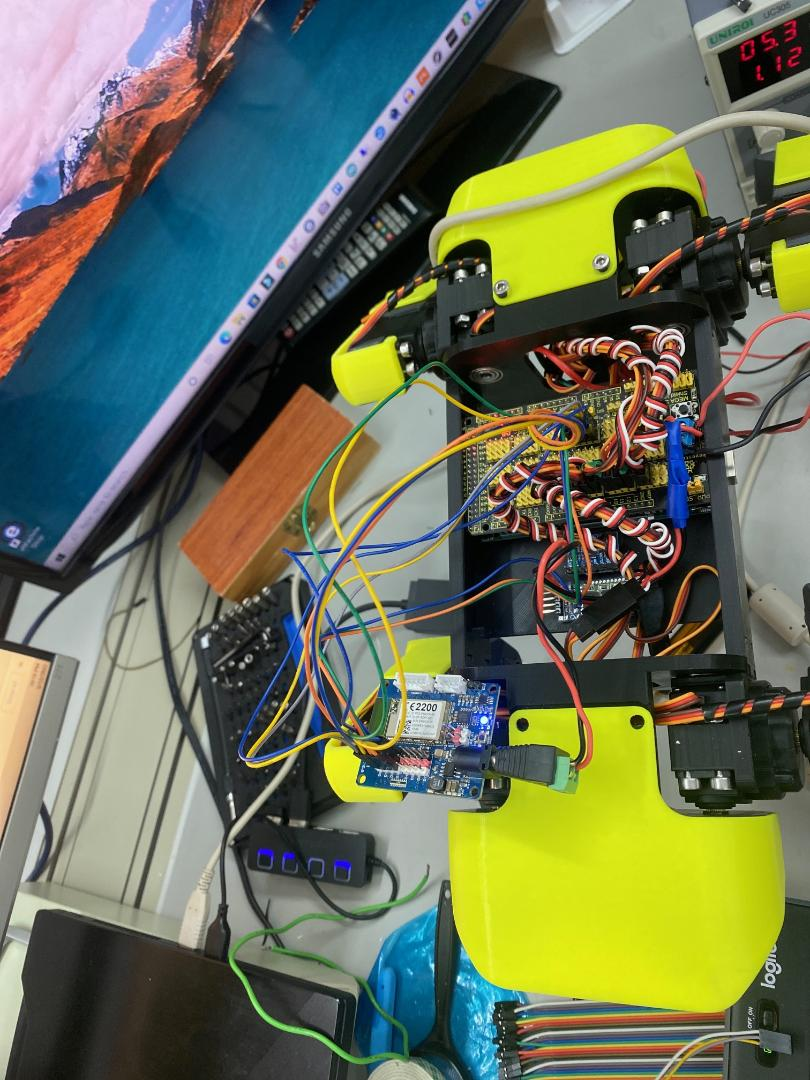#13
looks great.

Here is the code from my 19 DOF biped I used to make it walk.
`Code:no code`
oh yeah there isn’t any code because I used an EZB and a movement panel.#14
Nink,
Is your project code posted public ally on the cloud drive?
#15
Make sure you have a GND connected between the two

You send PWM with the pwm command (i'm guessing you are using ezscript): https://synthiam.com/Support/ez-script-api/ezscript

`Code:PWM (digitalPort, speed) Set the PWM (Pulse Width Modulation) to the desired duty percentage cycle This simulates voltage on the specified pin (Between 0 and 5v) PWM Value is between 0 and 100 Example: PWM(D14, 90)`
#16
DJ, I tried that.  But the arduino code must have been misbehaving.  Anyway, I booted the whole approach and moved right over the the EZBV4! Got the servo profiles setup and spot on his feet.

What tutorial do you recommend for using the frame approach for the servo motions?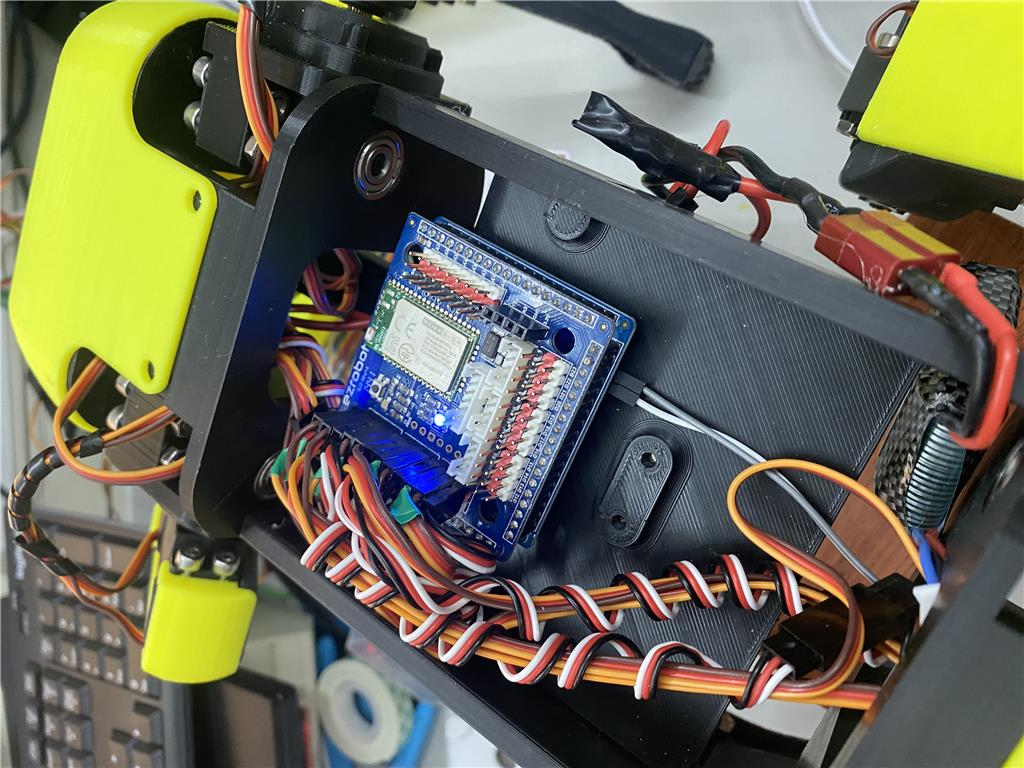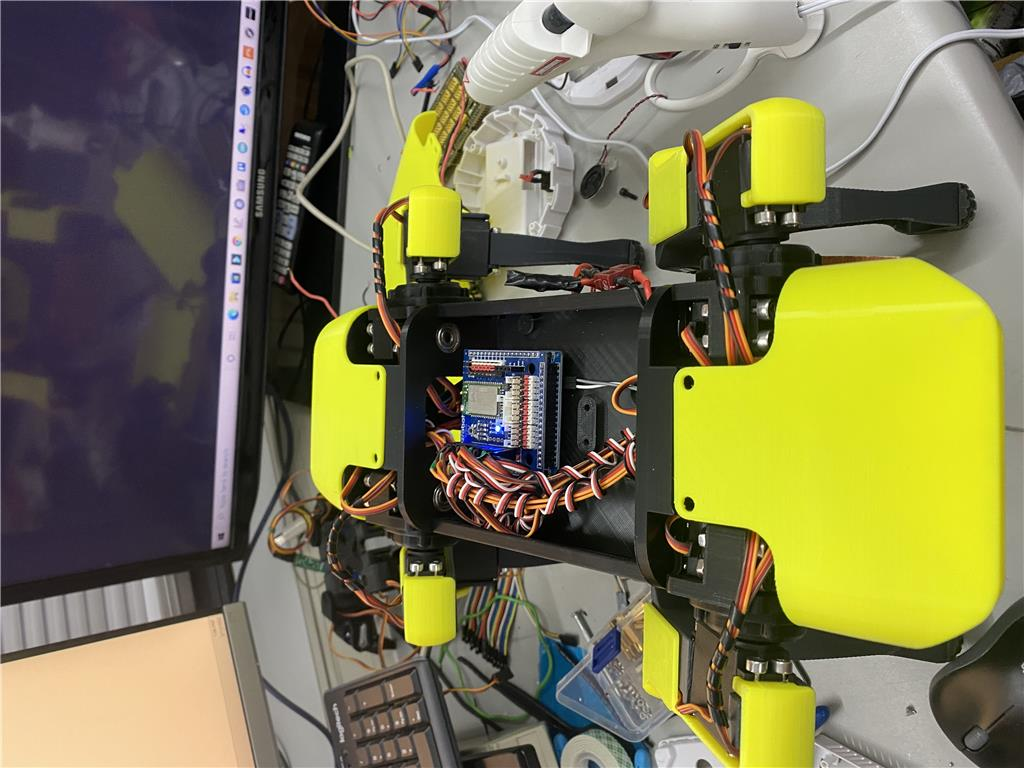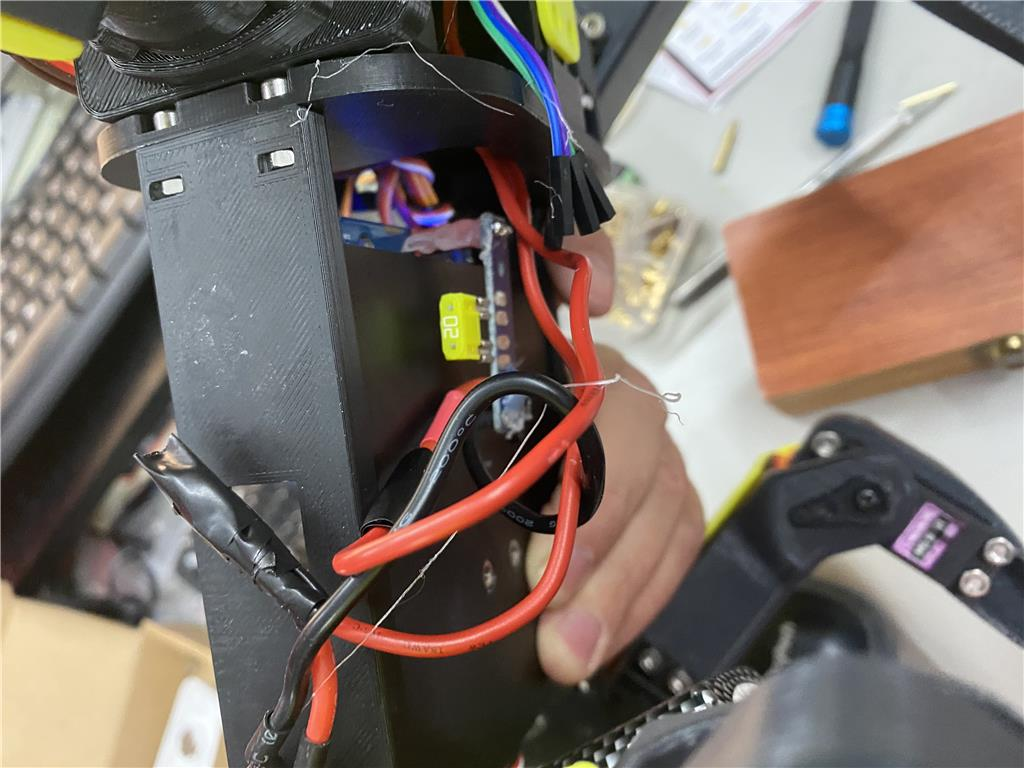#18
Looking at the code, all of the positions you need are in there. The value of 0 in their code would be 90 in arc. Because they use - negative values for one way and positive for the other
#19
That arduino code also has an IMU connected. Do you have one on the i2c port? Maybe that's why it isn't working for you. I'm guessing they use it for helping balance and such. Probably a good idea to use their code and control it via pwm from the iotiny if you can. That way you can build off the work they've already done rather than reinventing it.
PRO
USA
#21
I was wondering if this project is still active. I am considering making something like this.
#22
Please see my other comment in this in the other thread. If you are looking on ways to program this or your other quad, you may want to look here. Might find something with some research.